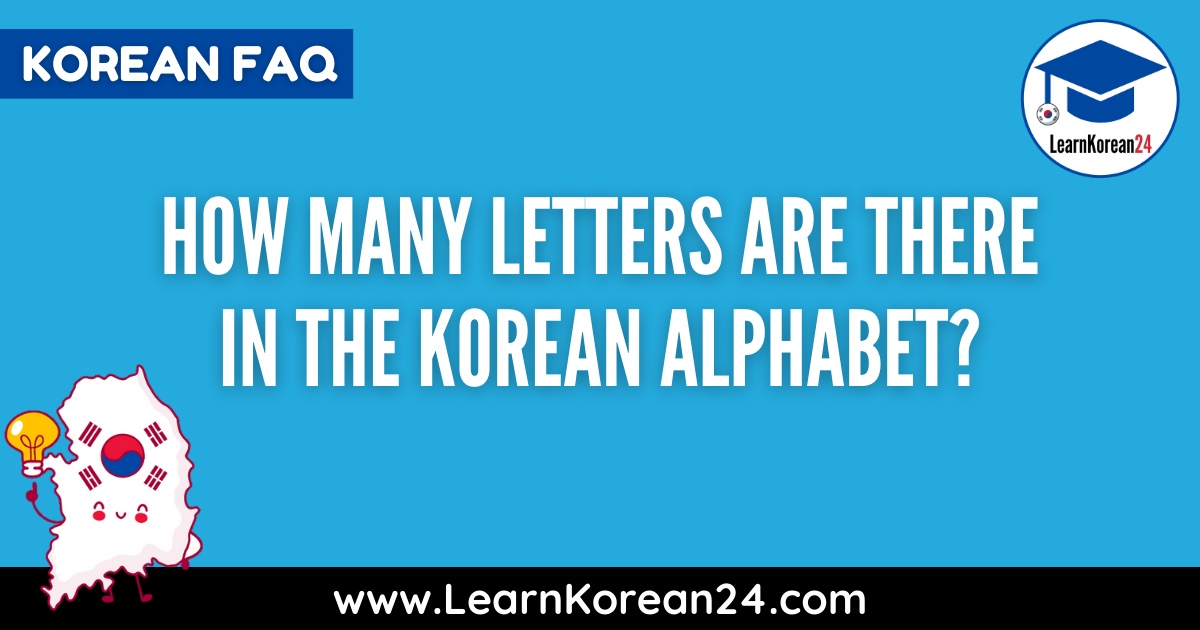# How Many Letters In The Korean Alphabet?The Korean alphabet, known as Hangul (한글), is one of the easiest foreign language alphabets to learn. If you’re interested in learning the Korean alphabet, you may be curious how many letters are in the Korean alphabet. In this short lesson, we will explain how many letters are in the Korean alphabet and the names of the Korean letters.

## How Many Letters In The Korean Alphabet?

The Korean Alphabet, known as Hangul, has 24 letters. The Korean alphabet is made up of 14 basic consonants and 10 basic vowels. In addition to this, the Korean alphabet also has 5 double consonants and 11 combined vowels.

## What Are The Korean Letter Names?

### Korean Consonant Names

The names of the 14 basic consonants in the Korean alphabet are as follows:

• = 기역 [gi-yeok]
• = 니은 [ni-eun]
• = 디귿 [di-geut]
• = 리을 [ri-eul]
• = 미음 [mi-eum]
• = 비읍 [bi-eup]
• = 시옷 [si-ot]
• = 이응 [i-eung]
• = 지읒 [ji-eut]
• = 치읓 [chi-eut]
• = 키읔 [ki-euk]
• = 티읕 [ti-eut]
• = 피읖 [pi-eup]
• = 히읗 [hi-eut]

### Korean Vowel Names

Next, the names of the 10 basic Korean vowels. Unlike the consonants above, the names of Korean vowels are simply the same as the sounds they make. The names of the 10 basic Korean vowels are as follows:

• = [a]
• = [ya]
• = [eo]
• = [yeo]
• = [o]
• = [yo]
• = [u]
• = [yu]
• = [eu]
• = [i]

### Korean Double Consonant Names

Korean double consonants are made by doubling a basic consonant. There are 5 double consonants. The names of the 5 Korean double consonants are as follows:

• = 쌍기역 [ssang-gi-yeok]
• = 쌍디귿 [ssang-di-geut]
• = 쌍비읍 [ssang-bi-eup]
• = 쌍시옷 [ssang-si-ot]
• = 쌍지읒 [ssang-ji-eut]

### Korean Combined Vowel Names

Korean combined vowels are made of a combination of 2 basic vowels. The names of the 11 Korean combined vowels are as follows:

• = [ae] ( +)
• = [yae] (+)
• = [e] (+)
• = [ye] (+)
• = [wa] (+)
• = [wae] (+)
• = [oe] (+)
• = [wo] (+)
• = [we] (+)
• = [wi] (+)
• = [ui] (+)